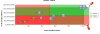Recommended Posts

Hello! I want to plot Boston Matrix with bubble chart.I calculate the minimum and maximum of the data and draw quadrant (attributes "drawQuadrant"=1 , "quadrantXVal"=(min+max)/2., etc.)

Now I need to paint sectors a different color. I use 2 trendlines ("isTrendZone" = 1), "startValue"=min, "endValue"=(min+max)/2. and "startValue"=(min+max)/2., "endValue"=max

But the plot gets out beyond the minimum and maximum.

Can I paint over sectors in the right way? Or it may be a different type of graph (not bubble)?

Thanks

bubble.xml